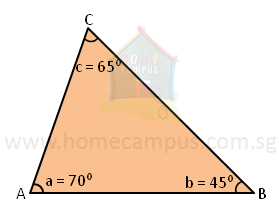## What is Angle Sum of a Triangle?

Practice Unlimited Questions

#### 1. Finding the sum of angles of a triangle

METHOD 1: Using a protractor

Make a triangle such as the one below. Then, using a protractor measure each of its angles.In the figure above,
∠a  =  70°
∠b  =  45°
∠c  =  65°

∠a  +  ∠b  +  ∠c  =  70°  +  45°  +  65°  =  180°
We notice that the angle sum of the above triangle is 180°.

Try making a couple of triangles, measure their angles and record their angle sums.
You will observe that in every case, the angle sum of a triangle is 180°.
METHOD 2: Using the hands-on method

Make a triangle as below. Then cut it into 3 pieces and arrange the pieces next to each other.Upon putting the pieces together you will notice that the 3 corners join together to form the sum of angles along a straight line which is 180°.

Conclusion:

The angle sum or sum of angles of a triangle is 180°.